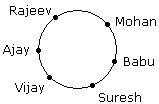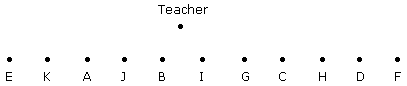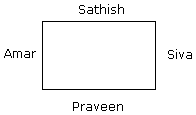# Tips to solve seating arrangement problems#### SEATING ARRANGEMENT

•   In order to solve seating arrangement questions, first of all diagram should be made. By doing so questions are easily and quickly solved.

Example 1:

1. 6 Boys are sitting in a circle and facing towards the centre of the circle.
2. Rajeev is sitting to the right of mohan but he is not just at the left of Vijay.
3. Suresh is between Babu and Vijay.
4. Ajay is sitting to the left of Vijay.

Who is sitting to the left of Mohan ?

Solution :Hence, Babu is sitting to the left of Mohan.

Example 2:

1. Eleven students A, B, C, D, E, F, G, H, I, J and K are sitting in first line facing to the teacher.
2. D who is just to the left of F, is to the right of C at second place.
3. A is second to the right of E who is at one end.
4. J is the nearest neighbour of A and B and is to the left of G at third place.
5. H is next to D to the right and is at the third place to the right of I.

Who is just in the middle ?

Solution :Hence, I is just in the middle.

Example 3:

Siva, Sathish, Amar and Praveen are playing cards. Amar isto the right of Sathish, who is to the right of Siva.

Who is to the right of Amar ?

Solution :Hence Praveen is to the right of Amar.

Example 4:

1. A, B and C are three boys while R, S and T are three girls. They are sitting such that the boys are facing the girls.
2. A and R are diagonally opposite to each other.
3. C is not sitting at any of the ends.
4. T is left to R but opposite to C.

(A). Who is sitting opposite to B ?

(B). Who is sitting diagonally opposite to B ?

Solution :(A). Hence, R is sitting opposite to B.

(B). Hence, S is sitting diagonally opposite to B.

• N! = N(N-1)(N-2)(N-3)……1
•  0! = 1! = 1
•  nCr = n!/( n−r) !( r!)
•  nPr = n! /(n−r) !

▪ Arrangement : n items can be arranged in n! ways

▪ Permutation : A way of selecting and arranging r objects out of a set of n objects, nPr = n!/( n−r) !

▪ Combination : A way of selecting r objects out of n (arrangement does not matter) nCr = n! /(n−r )!(r!)

▪ Selecting r objects out of n is same as selecting (n-r) objects out of n, nCr = nCn-r

▪ Total selections that can be made from ‘n’ distinct items is given  ∑ k=0n nCk = 2 n

#### Partitioning :

▪ Number of ways to partition n identical things in r distinct slots is given by (n+r−1)C(r−1)

▪ Number of ways to partition n identical things in r distinct slots so that each slot gets at least 1 is given by (n−1)C(r−1)

▪ Number of ways to partition n distinct things in r distinct slots is given by r n

▪ Number of ways to partition n distinct things in r distinct slots where arrangement matters = (n+r−1)! /(r−1)!

#### Arrangement with repetitions :

• If x items out of n items are repeated, then the number of ways of arranging these n items is n!/ x! ways.
• If a items, b items and c items are repeated within n items, they can be arranged in n! /(a! b! c!) ways.

#### Rank of a word :

▪ To get the rank of a word in the alphabetical list of all permutations of the word, start with alphabetically arranging the n letters. If there are x letters higher than the first letter of the word, then there are at least x*(n-1)! Words above our word.

▪ After removing the first affixed letter from the set if there are y letters above the second letter then there are y*(n-2)! words more before your word and so on. So rank of word = x*(n-1)! + y*(n-2)! + .. +1

#### Integral Solutions :

▪ Number of positive integral solutions to x1+x2+x3+…..+xn= s where s ≥ 0 is  (s−1)C(n−1)

▪ Number of non-negative integral solutions to x1+x2+x3+…..+xn = s where s ≥ 0 is   (n+s−1)C(n−1)

#### Circular arrangement :

Number of ways of arranging n items around a circle are 1 for n = 1,2 and (n-1)! for n≥3. If its a necklace or bracelet that can be flipped over, the possibilities are (n-1)! /2

###### Derangements :

If n distinct items are arranged, the number of ways they can be arranged so that they do not occupy their intended spot is D = n!(1 /0! − 1/ 1! + 1 /2! − 1 /3! +…… ( −1 ) n /n! )

Correct! Wrong!

Correct! Wrong!

Correct! Wrong!

Correct! Wrong!

#### A, B, C, D and E are sitting on a bench. A is sitting next to B, C is sitting next to D, D is not sitting with E who is on the left end of the bench. C is on the second position from the right. A is to the right of B and E. A and C are sitting together. In which position A is sitting ?

Correct! Wrong!

seating arrangement
Fabulous!
Good work!
Better luck next time

Share your Results:

Don't miss out!
Subscribe To Our Newsletter

Learn new things. Get an article everyday.

Invalid email address
Give it a try. You can unsubscribe at any time.